Next: Evolution in the H-R Up: Local Star Formation Process Previous: Second Core   Contents

# Example of Numerical Simulation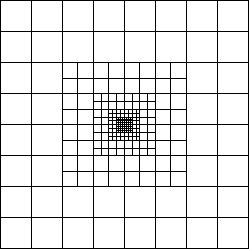We have described the cloud run-away collapse and succeeding accretion process In the latter phase, there is a possibility that the outflow is driven by the effect of magnetic Lorentz force. A restricted number of numerical simulation can simulate such evolution throughout. The size of the molecular cores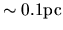is more than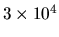times as large as the typical size of the first core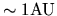. Therefore numerical scheme to calculate such process must have a large dynamic-range. In the finite-difference scheme, the spatial dynamic range is restricted by the number of the cells. Although at least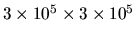grid points (in 2D) seems necessary to resolve a factor of, to solve the MHD equation with the Poisson equation of the self-gravity have not been done. This is done by the Eularean nested-grid simulation. This method uses a finite number of grid systems with different spatial resolutions are prepared. Coarse grid covers whole the cloud and is to see a global structure. Fine grid covers only the central part of the cloud and is to see the fine-scale structure appeared near the center. Figure 4.14 explains grid cells of the nested grid method. The size of the cells of the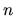-th level grid (L) is taken equal to a half of those of the L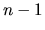.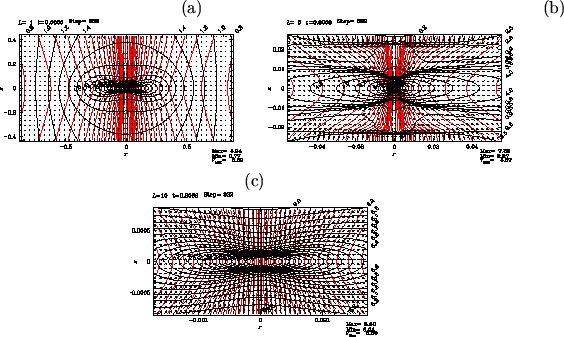A cylindrical cloud with coaxial magnetic field in hydrostatic balance is studied. Rotation vector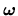and the magnetic field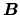are parallel to the symmetric axis of the cylinder (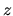-axis). For the energy equation, a double-polytropic equation of state is adopted, since the radiation hydrodynamical calculation (for example Masunaga & Inutsuka 2000) predicts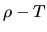relations like shown in Figure 4.12, which consists of a number of power-laws for some density ranges.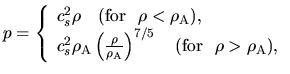(4.98)

where we take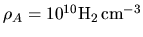. Figure 4.15 is the model of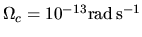,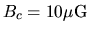,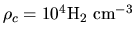,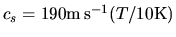, and scale-length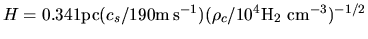. This is a snapshot of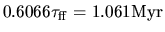. The cylindrical cloud breaks into pieces with a length in-direction equal to the most unstable wavelength of the gravitational instability (Matsumoto, Nakamura, & Hanawa 1994) as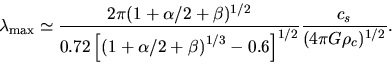(4.99)

The magnetic fields prevent gas motions perpendicular to the field lines. Further, the centrifugal force works in the perpendicular direction to. A disk forms which running in the perpendicular direction toandand thus to the-axis. Figure 4.15 illustrates a snapshot at the time of. Level 1 grid (upper-left) captures the global structure of the contracting disk perpendicular to the-axis. Close up view is shown in L5, which has 16 times finer spatial resolution (upper right). Since speed,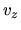, of infalling gas is larger than the sound speed, a number of shock fronts are formed near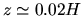and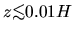. Further inner structure is represented in L10 grid (bottom) which has 32 times finer resolution than L5. Another discontinuity in density is now forming near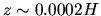. Since gas inside of this discontinuity has the density larger than the critical density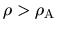, in this phase the first core (p.) begins to be formed. In the isothermal collapse phase, a characteristic power-law for the Larson-Penston self-similar solution is seen in the radial distributions of density as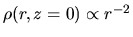, and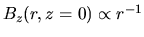.

After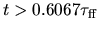the first core is formed. Radial infall motion is accelerated toward the surface of the first core and the rotational motion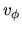also increases. This amplification promotes the toroidal magnetic field,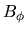. Since the torque is proportional to, the amplitude of the torque increases after the first core formation.

Structure just after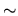500 yr has passed from Figure 4.15 is shown in Figure 4.15 In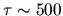yr from the epoch of Figure 4.15, flow is completely changed and outflow is launched. Panel (b), which corresponds to a four-times close-up of panel (a), shows clearly that outflow is ejected from a region near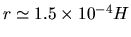and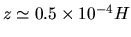. And the outflow vectors and the disk has an gngle of approximately 45 deg. The magneto-centrifugal wind model favors a small angle between the magnetic field and the disk,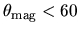deg. This model predicts that the angle between the flow and the disk is also smaller than 60 deg. Numerical results is not inconsistent with this prediction of magneto-centrifugal wind model.

To see which force is driving such outflow, amplitudes of three forces are compared: the Lorentz force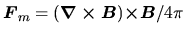, the centrifugal force, and the thermal pressure gradient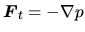. The parallel components to the poloidal magnetic field of the three forces are as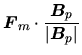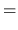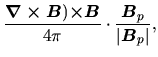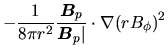(4.100)

(Ustyugova et al. 1999)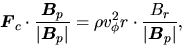(4.101)

and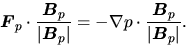(4.102)

Figure 4.16 illustrates the largest force at each grid point. Centrifugal force dominated region (region C), which is shown by asterisk (*), overlaps the outflow region around point P1 (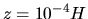,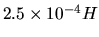). Just radially exterior to this region C, there is another region near point P2 (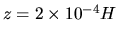,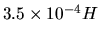) filled with puls signs where the magnetic force is dominated (region M). Comparing with the flow vectors in the left, the strong outflow coincides with this region M and the above region C. Therefore, the outflow is driven by the centrifugal force and the Lorentz force (pressure gradient by toroidal magnetic fields).

Difference between models with different magnetic field strength but the same rotation speed is illustrated in Figure 4.17. Magnetic to thermal pressure ratio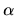is taken 1 [panels (a), and (d)], 0.1 [panels (b), and (e)], and 0.01 [panels (c), and (f)]. Panels (a), (b), (c) in the left column are snapshots when the central density reaches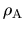while panels (d), (e), (f) in the right column show the structure after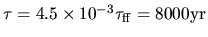passed. In the model with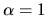, the outflow gas flows through a region whose shape resembles a capital letter U. This is similar to that observed in model (and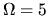) shown in Figure 4.16. Decreasing the magnetic field strength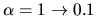and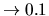, the shape of disk changes from a flat disk to a round ellipsoid. The model with neither magnetic field nor rotation shows a spherical contracting core. Outflow is also affected by decrease in magnetic field strength. Globally folded magnetic field lines are seen in the outflow region in panel (e). Smaller-scale structure folding the magnetic field lines develops in the outflow region in panel (f). The strucure seen in the polidal magnetic field is well correlated to the distribution of toroidal magnetic field strength. That is, a packet of strongpinch the gas and poloidal magnetic field lines radially inwardly. This means that the toroidal magnetic field is amplified by the rotation motion and becomes predominant over the poloidal one in the case with meak initial magnetic field. Thus, the whoop stress, which is expressed by the first term of the Lorentz force of equation(B.13) and comes from the tension of toroidal magnetic field, pinches the plasma contained inside of the loop of. For this case, the magnetic force dominant region is widely spread compared with the centrifugal force dominant region, which is far from the model with strong magnetic field. In this case, the magnetic pressure gradient plays an important role. Gas of outflow is ejected perpendicularly from the disk and forms flow similar to the capital letter I. In conclusion, U-type and I-type outflows are generated for strong magnetic model and weak magnetic model, respectively.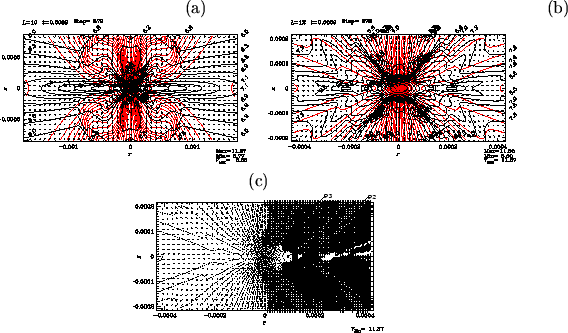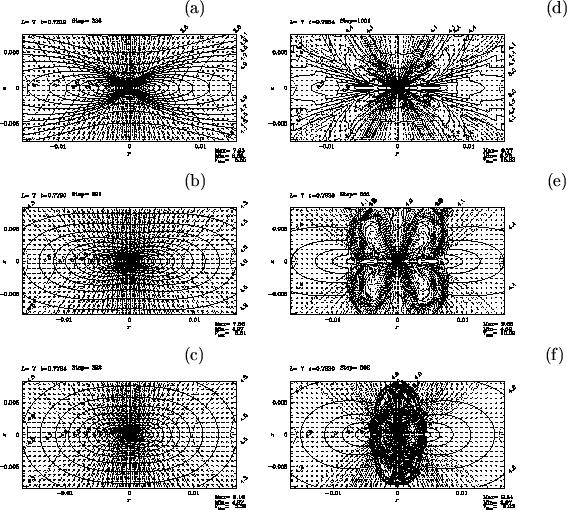Next: Evolution in the H-R Up: Local Star Formation Process Previous: Second Core   Contents
Kohji Tomisaka 2007-07-08